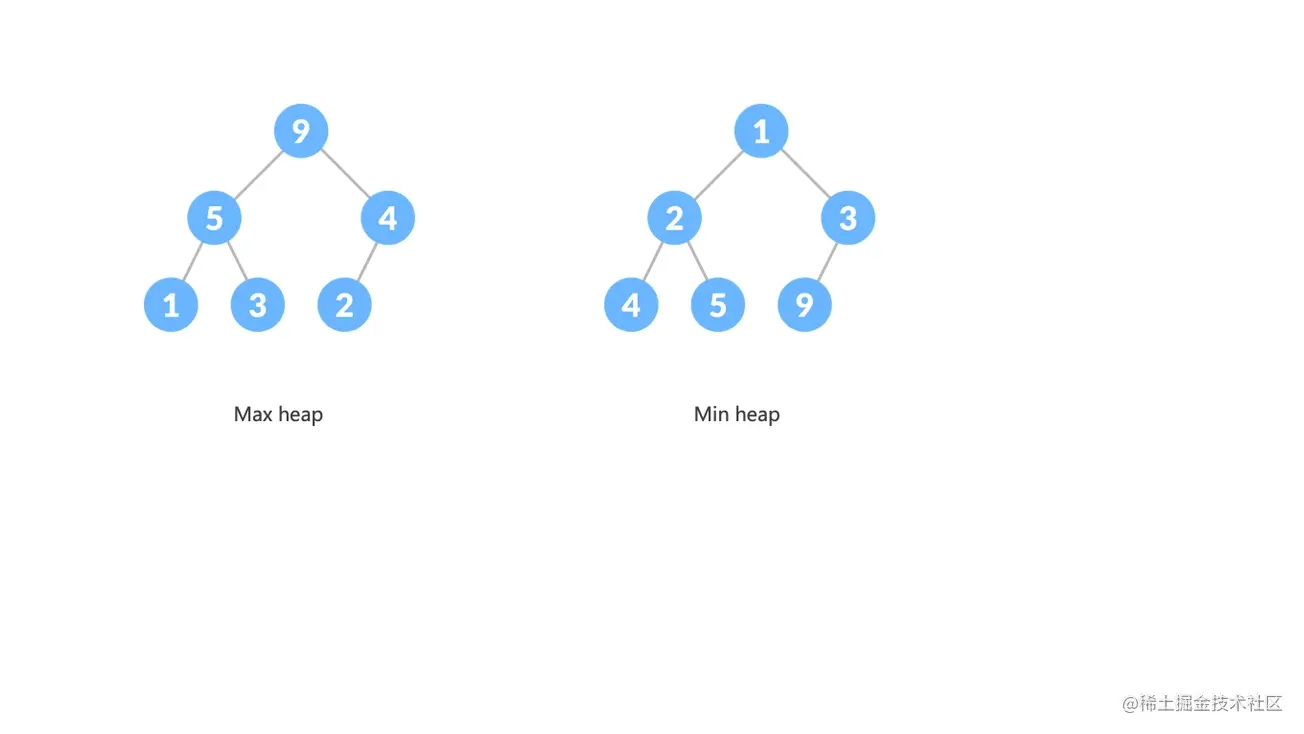﻿ Javascript数据结构之栈和队列详解_javascript技巧_脚本之家
javascript技巧# Javascript数据结构之栈和队列详解

## 前言

### 栈（stack）### 栈实现

• push（插入新元素，并让新元素成为栈顶元素）
• pop（栈顶元素出栈，并返回栈顶元素）
• peek（想知道栈最后添加的是哪个，用这个方法。返回栈顶元素，不出栈。是个辅助方法）
• clear（清空栈）
• isEmpty（若栈为空，返回 true，否则返回 false）
• size（返回栈元素个数）
```class Stack {
constructor() {
this.items = [];
}
push(item) {
this.items.push(item);
}
pop() {
return this.items.pop();
}
peek() {
return this.items[this.items.length - 1];
}
clear() {
this.items = [];
}
isEmpty() {
return this.items.length === 0;
}
size() {
return this.items.length;
}
}
const stack = new Stack();
stack.push('c++');
stack.push('swift');
stack.push('python');
stack.push('javascript');
console.log(stack.isEmpty()); // false
console.log(stack.size());    // 4
console.log(stack.peek());    // javascript
const removedItem = stack.pop();
console.log(removedItem);     // javascript
console.log(stack.peek());    // python
stack.clear();
console.log(stack.isEmpty()); // true
console.log(stack.size());    // 0```

### 解决实际问题

```function transitionToBin(decNumber) {
const stack = new Stack();
do {
// 每次循环计算出的低位值，依次入栈
stack.push(decNumber % 2);
decNumber = Math.floor(decNumber / 2);
} while(decNumber > 0);
let result = '';
// 此时，stack 中存放的是转换后二进制值，栈顶是高位，依次向下。
while (stack.size() > 0) {
// 从栈顶的高位依次出栈，拼接到显示结果中
result += stack.pop();
}
return result;
}
const binNumber = transitionToBin(321);
console.log('binNumber: ', binNumber);```

### 栈的另外应用

```function factorial(n) {
return n > 1 ? n * factorial(n - 1) : n;
}
console.log(factorial(4));```## 简单队列（Queue）### 队列实现

• enqueue 把一个新元素推入队列
• dequeue 从队列中移除一个已有元素

```class Queue {
constructor() {
this.items = [];
}
// 推入
enqueue(item) {
this.items.push(item);
}
// 移除
dequeue() {
return this.items.shift();
}
// 队列头元素
peek() {
return this.items;
}
// 为空判断
isEmpty() {
return this.items.length === 0;
}
size() {
return this.items.length;
}
}```

```const treeData = {
node: {
value: 12,
children: [{
value: 30,
children: [{
value: 22,
children: null
}, {
value: 10,
children: [{
value: 5,
children: null
}, {
value: 4,
children: null
}]
}]
}, {
value: 6,
children: [{
value: 8,
children: null
}, {
value: 70,
children: null
}]
}]
}
};```

```function bfs(tree) {
// 准备一个空的队列
const queue = new Queue();
queue.enqueue(tree);
// 一个用于显示结果的数组
const result = [];
do {
// 出队列
let node = queue.dequeue();
result.push(node.value);
if (node.children && node.children.length > 0) {
node.children.forEach(sub => {
queue.enqueue(sub);
});
}
} while (queue.size() > 0);
// 显示遍历结果
console.log('result:', result.join(', '));
}
bfs(treeData.node);
// result: 12, 30, 6, 22, 10, 8, 70, 5, 4```## 优先队列

• 医院对病人的分诊，重症的优先给予治疗
• 我们销售某件商品时，可以按照该商品入库的进货价作为条件，进货价高的优先拿出销售。

### 优先队列实现

#### 线性数据结构实现优先队列

• 第一种，就是插入的时候，不考虑其他，就在队列末尾插入。而移除的时候，则要根据优先级找出队列中合适的元素移除。
• 第二种是，插入元素的时候，根据优先级找到合适的放置位置，而移除的时候，直接从队列前面移除。

```class QItem {
constructor(item, priority) {
this.item = item;
this.priority = priority;
}
toString() {
return `\${this.item} - \${this.priority}`;
}
}
class PriorityQueue {
constructor() {
this.queues = [];
}
// 推入
enqueue(item, priority) {
const q = new QItem(item, priority);
let contain = false;
// 这个队列本身总是按照优先级，从大到小的
// 所以找到第一个比要插入值小的那个位置
for (let i = 0; i < this.queues.length; i++) {
if (this.queues[i].priority < q.priority) {
this.queues.splice(i, 0, q);
contain = true;
break;
}
}
// 都比它大，放最后
if (!contain) {
this.queues.push(q);
}
}
// 移除
dequeue() {
return this.queues.shift();
}
// 队列头元素
peek() {
return this.queues;
}
isEmpty() {
return this.queues.length === 0;
}
size() {
return this.queues.length;
}
}
const queue = new PriorityQueue();
queue.enqueue('K40', 3100);
queue.enqueue('K50', 5000);
queue.enqueue('K10', 6100);
queue.enqueue('K10', 6000);
queue.enqueue('K10', 5600);
queue.enqueue('K50', 4600);
queue.enqueue('K40', 5900);
console.log(queue.dequeue());
console.log(queue.dequeue());
console.log(queue.dequeue());
console.log(queue.dequeue());
console.log(queue.dequeue());
console.log(queue.dequeue());
console.log(queue.dequeue());
/*
QItem { item: 'K10', priority: 6100 }
QItem { item: 'K10', priority: 6000 }
QItem { item: 'K40', priority: 5900 }
QItem { item: 'K10', priority: 5600 }
QItem { item: 'K50', priority: 5000 }
QItem { item: 'K50', priority: 4600 }
QItem { item: 'K40', priority: 3100 }
*/```

#### Heap（堆）数据结构实现优先队列

• 它是一个完全二叉树（complete binary tree） 的数据结构。所谓完全二叉树（complete binary tree）,就是整个二叉树，除了底层的叶子节点，其他的层都是填满的，而且底层的叶子节点，从左到右不能有空的。（这样一个完全二叉树就能使用 Array 这种线性结构来存储）
• 大顶堆（Max heap） ：父节点的值大于或者等于子节点的值，堆顶是这个堆的最大元素
• 小顶堆（Min heap） ：父节点的值小于或者等于子节点的值，堆顶是这个堆的最小元素#### 代码实现一个二叉堆

• 新元素一律先插入到堆的尾部
• 依次向上调整整个堆的结构（一直到根即可）

HeapifyUp• 取出顶部元素（因为它永远是最大那个）
• 将尾元素替换到顶部（先不用管它的大小）
• 依次从根部向下调整整个堆的结构（一直到堆尾即可）

HeapifyDown```class Heap {
constructor(comparator = (a, b) => a - b) {
this.arr = [];
this.comparator = (iSource, iTarget) => {
const value = comparator(this.arr[iSource], this.arr[iTarget]);
if (Number.isNaN(value)) {
throw new Error(`Comparator should evaluate to a number. Got \${value}！`);
}
return value;
}
}
enqueue(val) {
// 插入到末尾
this.arr.push(val);
// 向上冒泡，找到合适位置
this.siftUp();
}
dequeue() {
if (!this.size) return null;
const val = this.arr.shift();
const rep = this.arr.pop();
this.arr.splice(0, 0, rep);
this.siftDown();
}
get size() {
return this.arr.length;
}
siftUp() {
// 新元素索引
let index = this.size - 1;
// 根据完全二叉树的规则，这里我们可以依据元素索引index的值，获得他对应父节点的索引值
const parent = (i) => Math.floor((i - 1) / 2);
if (parent(index) >= 0 && this.comparator(parent(index), index) < 0) {
// 如果父节点存在，并且对比值比当前值小，则交互位置
this.swap(parent(index), index);
index = parent(index);
}
}
siftDown() {
let curr = 0;
const left = (i) => 2 * i + 1;
const right = (i) => 2 * i + 2;
const getTopChild = (i) => {
// 如果右节点存在，并且右节点值比左节点值大
return (right(i) < this.size && this.comparator(left(i), right(i)) < 0)
? right(i) : left(i);
};
// 左节点存在，并且当前节点的值，小于子节点中大的那个值，交换
while (left(curr) < this.size && this.comparator(curr, getTopChild(curr)) < 0) {
const next = getTopChild(curr);
this.swap(curr, next);
curr = next;
}
}
// 交换位置
swap(iFrom, iTo) {
[this.arr[iFrom], this.arr[iTo]] = [this.arr[iTo], this.arr[iFrom]];
}
}
const heap = new Heap();
heap.enqueue(56);
heap.enqueue(18);
heap.enqueue(20);
heap.enqueue(40);
heap.enqueue(30);
heap.enqueue(22);
console.log('heapify: ', heap.arr.join(', '));
heap.dequeue();
console.log('step 1: ', heap.arr.join(', '));
heap.dequeue();
console.log('step 2: ', heap.arr.join(', '));
heap.dequeue();
console.log('step 3: ', heap.arr.join(', '));
// heapify:  56, 40, 22, 18, 30, 20
// step 1:  40, 30, 22, 18, 20
// step 2:  30, 20, 22, 18
// step 3:  22, 20, 18```

#### 小顶堆在 React Scheduler 事务调度的包应用

Scheduler 存在两个队列，timerQueue（未就绪任务队列） 和 taskQueue（就绪任务队列）。当有新的未就绪任务被注册，就会 push 到 timerQueue 中，并根据开始时间重新排列任务顺序。

push 方法是在一个叫 schedulerMinHeap.js 的文件中基于最小堆（min-heap）来实现的。schedulerMinHeap 源码

```export function push(heap: Heap, node: Node): void {
const index = heap.length;
heap.push(node);
siftUp(heap, node, index);
}```

```function siftUp(heap, node, i) {
let index = i;
while (index > 0) {
const parentIndex = (index - 1) >>> 1;
const parent = heap[parentIndex];
if (compare(parent, node) > 0) {
// The parent is larger. Swap positions.
heap[parentIndex] = node;
heap[index] = parent;
index = parentIndex;
} else {
// The parent is smaller. Exit.
return;
}
}
}```